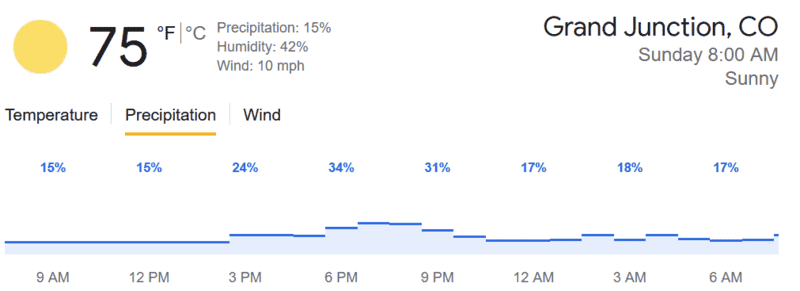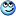# A chance of rain....

Staff Emeritus
TL;DR Summary
How does one interpret a chance of preciptitation as a probability?
What exactly does a chance of rain me? Tonight there is a 15% of rain. At one hour tonight, it's a 55% chance of rain. How exactly does this work out? How do I interpret these probabilities?

Interesting question. I have two ideas.

Clouds are noted by parts of 1, I think quarters, but it could also be eighth to inform pilots about the weather. So 3/4 clouds could translate to 75% probability of rain.

Or: there are clouds but it will not rain due to the terrain, only by a chance of 15% because it rained in 3 of 20 similar cases in the past.

Or a combination of both.

https://la.utexas.edu/users/kimmel/nwsforecasts.html said:
PRECIPITATION PROBABILITY

The probability of precipitation forecast is one of the most least understood elements of the weather forecast. The probability of precipitation has the following features:
... The likelihood of occurrence of precipitation is stated as a percentage
... A measurable amount is defined as 0.01" (one hundredth of an inch) or more
(usually produces enough runoff for puddles to form)
... The measurement is of liquid precipitation or the water equivalent of frozen precipitation
... The probability is for a specified time period (i.e., today, this afternoon, tonight, Thursday)
... The probability forecast is for any given point in the forecast area

To summarize, the probability of precipitation is simply a statistical probability of 0.01" inch of more of precipitation at a given area in the given forecast area in the time period specified. Using a 40% probability of rain as an example, it does not mean (1) that 40% of the area will be covered by precipitation at given time in the given forecast area or (2) that you will be seeing precipitation 40% of the time in the given forecast area for the given forecast time period.

Let's look at an example of what the probability does mean. If a forecast for a given county says that there is a 40% chance of rain this afternoon, then there is a 40% chance of rain at any point in the county from noon to 6 p.m. local time.

This point probability of precipitation is predetermined and arrived at by the forecaster by multiplying two factors:

Forecaster certainty that precipitation will form or move into the area
X
Areal coverage of precipitation that is expected
(and then moving the decimal point two places to the left)

Using this, here are two examples giving the same statistical result:
(1) If the forecaster was 80% certain that rain would develop but only expected to cover 50% of the forecast area, then the forecast would read "a 40% chance of rain" for any given location.
(2) If the forecaster expected a widespread area of precipitation with 100% coverage to approach, but he/she was only 40% certain that it would reach the forecast area, this would, as well, result in a "40% chance of rain" at any given location in the forecast area.

Yes, but...

If the probability of it raining in a night is 15%, how can the probability that it be in a subset of time - say 8PM to 9PM - be higher?

It must be correct...our Tech Overlords have approved it!••Hamiltonian, hutchphd and fresh_42
they may actually control the weather...

•Oh, and it's not just here. I picked a few cities at random (sort of - based on the forecast - looking at this for e.g. Death Valley would be kind of pointless) and this is not unusual, although my example is fairly extreme. But 25% chance of rain tonight and 30% between midnight and 1 AM is not at all unusual).

they may actually control the weather...
Sure they do!•Feel free to merge.

After reading that, though, I am still puzzled. Let's ignore correlations for the moment- but we know they are there. If we have a 20% probability of rain between 9 and 9 and between 1 and 3, and zero at all other times, the probability of rain overnnight should be 36%. I figure that's what they must be doing: making the hourly predictions and combining them (but including correlations and anticorrelations) to get the overall probability for that night. (Or day, or week, or whatever)

But that's clearly not what they are doing: today's forecast for Grand Junction, Colorado is a 15% chance of rain (all numbers from Google). But individual hours are more than twice that.Indeed, no single hour is below the overall probability. I see no way to combine the 8 trihourly estimates to get the overall probability. No matter what the correlations are.

You could say that the 15% is teh probability right this second, and not over the day, but you can find other cities where this isn't the case.

I think the question is on how to interpret this probability, not on how it is arrived at.
So my view is that there is only one way to interpret this probability, since it is not a recurring event (like tossing a coin): the notion of subjective probability based on games of chance. This is measured by imagining an urn with a mix of black/white balls that add to 100. Now a probability of say 15% of raining tomorrow, means you will prefer to bet on drawing a white ball out of an urn that contains 16 white balls and 84 black ones, rather than on raining tomorrow. Similarly for all percentages.

Maybe it's the probability that someone encounters rain during the time period.

Take an extreme case: a 12-hour period where there is 1 hour with 100% and 11 hours with 0%. The probability you encounter rain during this time period would be 100% / 12 = 8%. With the opposite case, (11 hours with 100% and 1 hour with 0%), you get a 92% probability for the whole period. I can plan for outdoor activities in the first case, but not so much with the second.

Summary: How does one interpret a chance of preciptitation as a probability?

What exactly does a chance of rain me? Tonight there is a 15% of rain. At one hour tonight, it's a 55% chance of rain. How exactly does this work out? How do I interpret these probabilities?
My understanding is the % chance or rain or probability is based on the expected/predicted fraction of the area to received precipitation. However,

This point probability of precipitation is predetermined and arrived at by the forecaster by multiplying two factors:
Forecaster certainty that precipitation will form or move into the area
X
Areal coverage of precipitation that is expected (and then moving the decimal point two places to the left).

https://www.weather.gov/media/pah/WeatherEducation/pop.pdf

During the last two weeks, our area has had probabilities of precipitation of 30 to 50%, but our location did not get rain. Instead, we've noticed rain around us, but not over us. The area had scattered, isolated thundershowers, most the time. We eventually did get two rain showers during two successive afternoons.

Areal coverage of precipitation that is expected (and then moving the decimal point two places to the left).
But this does not solve @Vanadium 50 riddle does it? A longer time interval will always produce at least as high a percentage (I believe that is the crux of the argument) Something funny here.
Perhaps there is always a rolling time average?...I sure don't see it

Maybe it's the probability that someone encounters rain during the time period.
That would mean a high probability for a long period and a low probability for a short period.

Something funny here.
I am guessing that there are two different sources here, or one source that is processed differently. For example, if the hour-by-hour forecast is updated hourly and the day/night forecaset is updated twice a day.

That would mean a high probability for a long period and a low probability for a short period.

What I meant was if you ask "What is the probability that it will rain this summer?" The true answer would be 100%. (It ought to rain sometimes, right?) But that would be a very useless information.

But if someone answered, say, 25%, one could imagine there will be 3 weeks of sunshine for every week of rain this summer. Or it could be 3 days for one day. And if it was 3 hours for each hour, the 25% prediction would still be true even though it will be raining every day this summer.

Of course, for that week, day, or hour that it is raining, a probability of 100% would be correct.

•anorlunda
Regarding @Vanadium 50 riddle, what time period does 15% refer to ; It is not clear.

If you go to the day-by-day view (on my phone), it says the probability of precipitation for Sunday is 15%.

Which I take to mean that should I call you at random times during the day and ask "is it raining" then 15% of the time yu will answer "yes" (on average).
I can also ask you "did it rain during the preceding hour" and that number can be as high as you wish (it could rain 10 minutes at the beginning of every hour.
So that works, right?

That interpretation would say that the probability of rain is always between the highest and lowest in that period. That's hard to reconcile.

I believe they are reporting two different averages.
1. The instantaneous "precip (15% in your forecast)" I take to mean that should I call you at random times during the day and ask "is it raining" then 15% of the time you will answer "yes" This is not the same as "will there be any rain during the 12 hr period
2. The hourly reports are the probability that sometime during that hour there will be rain (this is called PoP1hr I think). It need rain for only a shortl period of time
The answer to (1) is only very lightly constrained by (2) in this case.. In particular 0% would constrain all. But short intervals of rain hourly will produce your forecast numbers. This is surmise on my part...I don't do weather.

•Motore
jack action said:
https://la.utexas.edu/users/kimmel/nwsforecasts.html
PRECIPITATION PROBABILITY

The probability of precipitation forecast is one of the most least understood elements of the weather forecast
I think this sums things up pretty well...Last edited: